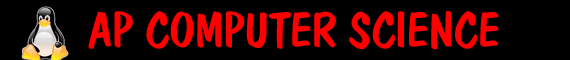Methods   We want to create a class called DiceAction that will be the most useful class of dice ever: It will have the following methods: For full credit, Math.random will be used once in rollDie(int sides) rollDie which will take in nothing and return the result of rolling a regular die rollDie which will take in an int for the numOfSides and return the result of rolling that die rollDice which will take in nothing and return the result of rolling 2 regular dice rollDice (taking in an int for the number of regular dice you want to roll and returns the result rollDice (take in 2 ints, one for how many dice and the other for how many sides each die has). it will return the total avgRoll (takes in nothing and returns the average of 10 regular die - could be decimals) avgRoll (takes in an int for how many dice and then returns the average of that many regular die - could be decimals) avgRoll (take in 2 ints, one for how many dice and the other for how many sides each die has and returns the average of those die - could be decimals) isEven (takes in nothing and returns true or false, if the regular die was even isEven (taking in 1 number, for how many sides the die has and returns if the random roll was even) Extra Credit: public char scattegories() // that will return a lower case letter randomly (look at pauls response, and ascii tables) public String scattegories(int length) // that will return a word with length letters where every letter is random and and every other letter is a vowel Make calls to your other methods to cut down on your coding time. To test use this code below: ``` public class DiceAction { //ALL YOUR METHODS GO HERE!!! public static void main(String[] args) { DiceAction myDice=new DiceAction(); //System.out.println("RollDie returns " + myDice.rollDie()); //System.out.println("RollDie(sides) returns " + myDice.rollDie(20)); //System.out.println("rollDice() returns " + myDice.rollDice()); //System.out.println("rollDice(5) " + myDice.rollDice(5)); //System.out.println("rollDice(5,8) " + myDice.rollDice(5,8)); //System.out.println("avgRoll returns " + myDice.avgRoll()); //System.out.println("avgRoll(100) returns " + myDice.avgRoll(100)); //System.out.println("avgRoll(100,10) returns " + myDice.avgRoll(100,10)); //System.out.println("isEven() returns " + myDice.isEven()); //System.out.println("isEven(5) returns " + myDice.isEven(5)); } } ```